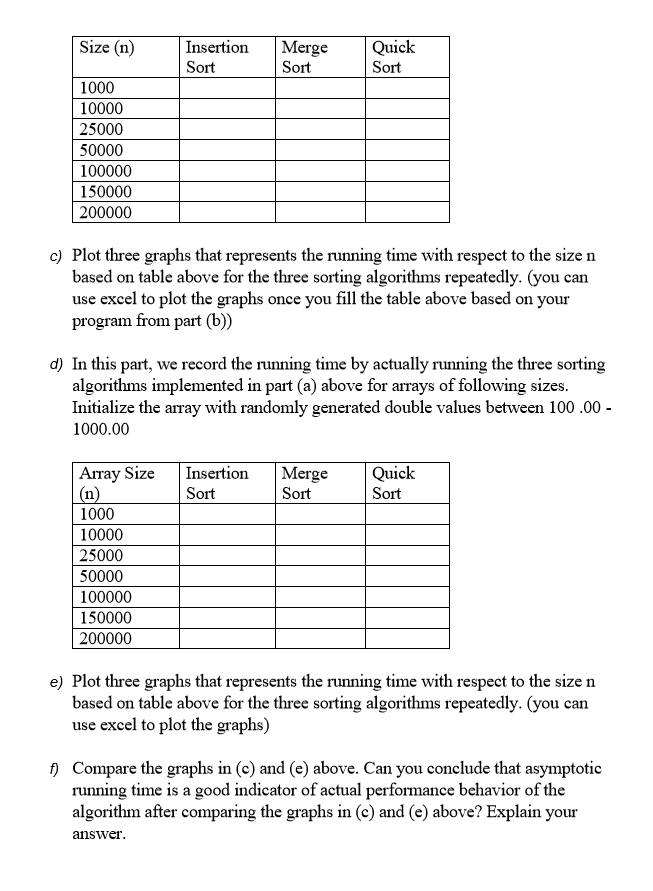# Answered! 1.160 Pts] Programming Question: In this problem you will be comparing the running time of merge sort, quick sort…1.160 Pts] Programming Question: In this problem you will be comparing the running time of merge sort, quick sort and selection sort algorithms a) Frist, develop three C++C methods that implement the above three sorting algorithms described in the book. Your code should match with the exact algorithms described in the book. I have provided a supplementary document with three sorting algorithms that you need to implement here. We will use these three methods in part (d) below b) Now write a C program that determines the running time based on asymptotic notation for above three sorting algorithms. Sorting Running time Tn) Algorithm Insertion Sort n nlog(n) Merge Sort Quick Sort nlog(n) Now run your program for n values given in the table and record the asymptotic notation based (theoretical) running time for all three soring algorithms

//Part a and b

#include <iostream>
#include <cstdlib>

Don't use plagiarized sources. Get Your Custom Essay on
Answered! 1.160 Pts] Programming Question: In this problem you will be comparing the running time of merge sort, quick sort…
GET AN ESSAY WRITTEN FOR YOU FROM AS LOW AS \$13/PAGE

using namespace std;

void selection_sort (int [], int);
void merge(int *,int, int , int );
void mergesort(int *a, int low, int high);
void quicksort (int [], int, int);

// Selection Sort
void selection_sort (int ar[], int size)
{
int min_ele_loc;
for (int i = 0; i < 9; ++i)
{
//Find minimum element in the unsorted array
//Assume it’s the first element
min_ele_loc = i;

//Loop through the array to find it
for (int j = i + 1; j < 10; ++j)
{
if (ar[j] < ar[min_ele_loc])
{
//Found new minimum position, if present
min_ele_loc = j;
}
}
//Swap the values
swap (ar[i], ar[min_ele_loc]);
}
}

void mergesort(int *a, int low, int high)
{
int mid;
if (low < high)
{
mid=(low+high)/2;
mergesort(a,low,mid);
mergesort(a,mid+1,high);
merge(a,low,high,mid);
}
return;
}
void merge(int *a, int low, int high, int mid)
{
int i, j, k, c;
i = low;
k = low;
j = mid + 1;
while (i <= mid && j <= high)
{
if (a[i] < a[j])
{
c[k] = a[i];
k++;
i++;
}
else
{
c[k] = a[j];
k++;
j++;
}
}
while (i <= mid)
{
c[k] = a[i];
k++;
i++;
}
while (j <= high)
{
c[k] = a[j];
k++;
j++;
}
for (i = low; i < k; i++)
{
a[i] = c[i];
}
}

void quicksort(int list[], int low, int high)
{
int pivot, i, j, temp;
if (low < high)
{
pivot = low;
i = low;
j = high;
while (i < j)
{
while (list[i] <= list[pivot] && i <= high)
{
i++;
}
while (list[j] > list[pivot] && j >= low)
{
j–;
}
if (i < j)
{
temp = list[i];
list[i] = list[j];
list[j] = temp;
}
}
temp = list[j];
list[j] = list[pivot];
list[pivot] = temp;
quicksort(list, low, j – 1);
quicksort(list, j + 1, high);
}
}

void random(int array[], int size){

for(int i=0; i<size; i++){
array[i] = (rand()%100)+1;
}
}

void display(int array[],int size){

for(int i=0; i<size; i++){
cout<<“n”<<array[i];
}
}
int main(){

int array;

random(array,1000);
display(array,1000);

selection_sort(array,1000);
display(array,1000);

mergesort(array,0,1000);
display(array,1000);

quicksort(array,0,1000);
display(array,1000);

return 0;
}